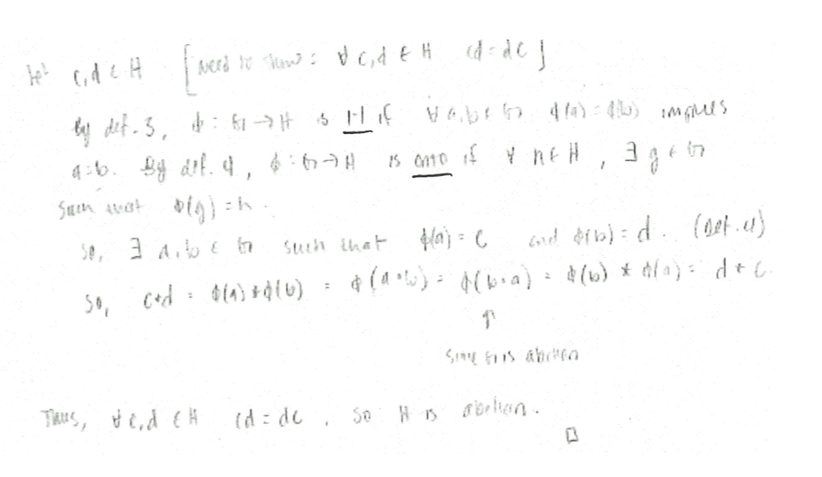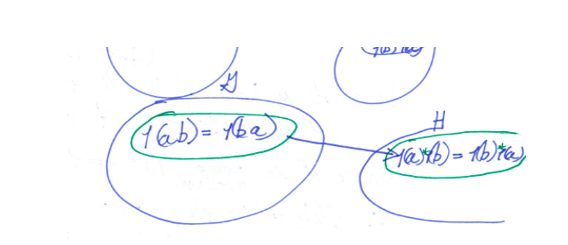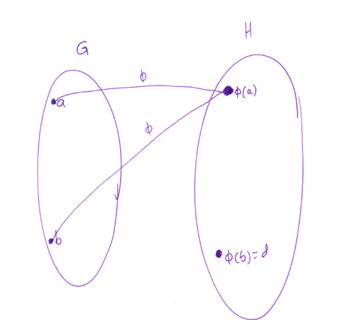# The Dysfunction of Functions in Abstract Algebra

Kathleen Melhuish & Kristen Lew
Texas State University

“[Functions] are completely different, which is what makes this course so challenging.” – Abstract Algebra Student

Functions are hard for students, even students in abstract algebra courses. Even if students have seen the definition and worked with examples of real functions throughout high school and college, their understanding might be stretched to a breaking point when it comes to ideas like homomorphisms on groups or rings. Fundamentally, we might know students don’t understand functions, but the extent to which they don’t understand functions goes deeper than we might think. In this blogpost, we will share some insights from our projects on key places where students’ idea of function can be detrimental to learning concepts of abstract algebra, and what we as instructors might be able to do about this.

The theme of our work is fortifying students’ understanding of functions while also forging through an abstract algebra curriculum. The issue of filling in gaps in prerequisite knowledge while still staying faithful to the current course is a challenge. We take on this challenge and hope that this post spurs others to take on this challenge with us, whether you teach abstract algebra or calculus, or any other course that relies on functions.

We have organized the post by projects led by the authors. We give an overview of each project and then describe what we have learned from each.

Project 1: The Group Theory Concept Assessment (GTCA)
Melhuish began developing this assessment as part of her dissertation, when she realized that there was no instrument that looked at student understanding of group theory (Melhuish, 2019). This assessment’s results, including over 1,000 responses from abstract algebra students over the past 5 years, as well as interviews with students about questions on the assessment, got us thinking: how do abstract algebra students think about function? Here’s one example. (And if this gets you curious as well, there are more examples in Melhuish and Fagan (2018).)

Function Understanding Thought 1: Abstract Algebra Students May Need a “Process” Understanding of Functions

Question (condensed): What is the kernel of ϕ(n)=i^n, ϕ:(Z,+)→(C,*)?

In total, fewer than 50% of students answered this question correctly. Many students, to our surprise, answered this item by providing a singleton element such as “{4}.” Yet, when we interviewed students about their responses, they typically provided an accurate definition that even contained language like “elements that map to the identity.”

We hypothesize that many abstract algebra students may still concieve of functions as “actions” (Breidenbach, Dubinsky, Hawks, & Nichols, 1992), meaning that they are not looking at a function holistically but rather element-by-element. Although this can helpful for direct computations such as “What is ϕ(4)?”, it is less helpful for finding preimages, particularly when the preimage has multiple elements (as kernels often do). For this, students may need to understand functions as a “process,” meaning that the function can be appreciated beyond acting on single elements. Students need to develop a process understanding to master early results in abstract algebra, such as the quotient struture of the First Isomorphism Theorem.

How might we address this? We suggest getting students to get their hands dirty with homomorphisms that are not one-to-one. Although students can recite the fact that a pre-image can have multiple elements, they might not have readily avaliable examples. After exploring examples, instructors can ask questions like, “How do you find all the elements that map to a particular location?” Students then reflect out loud and explicitly on how to generalize what they have explored. We also find it helpful to use relation diagrams during these discussions, such as those shown in Figure 1. Although these kinds of questions and diagrams can be used at many levels, including high school, we suggest that they are still worth taking up in advanced courses.

Project 2: Function Coherence in Abstract Algebra
As a next step, we interviewed a group of students to better understand how they connected functions in abstract algebra with their previous knowledge of functions in calculus and algebra. Our goal was to get a better understanding of their struggles to understand functions in an abstract setting (Melhuish, Lew, Hicks, & Kandasamy, 2019).

Function Understanding Thought 2: Abstract Algebra Students May Not Have a Mathematically Complete Definition of Function

We asked students: What is the definition of a function?

Across the six students we interviewed, only one could provide a complete definition for function (Student B). Here are the definitions the students gave:

Student A: A function is a relationship that maps members of the domain to a member of the range.
Student B: A function is a relation from one set to another where all the elements in the domain should be mapped to at most one element in the co-domain.
Student C: A function is a relation that takes an input and assigns it to exactly one output.
Student D: [An] equation that will do the same kind of operation to an input to get an output.
Student E: A function or a mapping takes a domain to a codomain following set rules.
Student F: A relation between two sets; can be one-to-one, onto, both, and/or neither.

When asked about their definitions, these students often appealed to the vertical line test (or in some cases, incorrectly, a horizontal line test.) However, in a setting like abstract algebra, this graph-based test can cause problems. When we graph a function, we study it analytically rather than algebraically.

How might we address this? We suggest not only stating the definition of function, but also getting students to talk about how this definition relates to maps in abstract algebra. Instructors can draw diagrams of homomorphisms, as well as show tables of homomorphisms, and help students see that one output is assigned to each input. By going back and forth between these diagrams and the formal definition of function, students have a better chance of seeing that the definition applies to abstract algebra.

Function Understanding Thought 3: Abstract Algebra Students May Not See Functions in Abstract Algebra as Functions

We asked students: Are the “functions” in abstract algebra the same as the functions in your prior mathematical experience?

While three students did think functions in abstract algebra were like prior functions, but with domains no longer limited to numbers, the other three students did not. One said, “What I thought of about function is always something to do with graph”; and another said that functions in abstract algebra don’t have a particular “rule.” These second three students—who focused on how different functions seemed in abstract algebra—struggled with the homomorphism tasks. Some believed that non-functions could still be homomorphisms. The first three students had more success with homomorphism tasks.

How might we address this? Here is a task we have found helpful: Which of the diagrams in Figure 1 could be a homomorphism? Which could be an isomorphism? Many students state that a diagram like the top-right could be a homomorphism (even though it is not a function.) This exploration could lead to discussion that both emphasizes the definition of function and explains that homomorphisms must themselves meet this requirement.Figure 1. Relation Diagrams. Each arrow points left to right, so these are diagrams of relations from G to H.

Project 3: Orchestrating Discussions Around Proof (Easier Said Than Done)

We are currently developing curriculum materials for abstract algebra instructors who want to facilitate deeper classroom discussions while still meeting coverage goals. You may have noticed similarities in our suggestions so far: talk about definitions and properties more explicitly, and use function diagrams. These suggestions show up throughout in our materials. In this section, we share some obstacles that emerged, and also say why these types of moves were ultimately productive.

To begin, we turn to students’ responses to a sample proof that isomorphisms preserve the property of being Abelian.Figure 2. One sample proof.

Function Understanding Thought 4: Explicitly Analyzing Proofs for Function Properties Can Be Powerful, but Students May Need to Disentangle the Function Definition (well-defined; everywhere defined) from Function Properties (1-1; onto)

We asked students, “Do we need a function to be one-to-one and onto in order for the Abelian property to be preserved? Where do we see these properties in the proof?” (Consider the sample proof in Figure 2.)

Initially, we wrote this question thinking that that students would recognize that, in this proof, the fact that an isomorphism is “onto” is critical, while being “one-to-one” is not. However, we found that students identified the need for onto, but also (incorrectly) argued that onto was also needed to show the existence of ϕ(g) of any g∈G. Later in the proof, the students identified claims like ab = ba implying ϕ(ab)=ϕ(ba) as a consequence of the function being one-to-one.

In conversations with the two groups of students who have tested this unit thus far, we have found that abstract algebra students have not disentangled everywhere defined and onto, and 1-1 and well-defined respectively. The properties of 1-1 and onto play substantial roles in a number of abstract algebra proofs. However, we rarely contrast them directly with function definition properties which may leave properties conflated.

How did we address this? We used the students’ claims about where 1-1 and onto were needed as a springboard to return to the definition of function, 1-1, and onto. This allowed students to discuss what well-defined (everywhere-defined) meant, and how well-defined differs from 1-1 (onto). Through these conversations, the students disentangled the properties and eventually arrived at the conclusion that the property of “onto” is needed, but 1-1 is not.

Function Understanding Thought 5: Function Diagrams Can Be Powerful, But Students May Need to Support to Understand and Leverage Them

We asked students: How can you represent the function in the proof (that isomorphism preserves the Abelian property) using a diagram?

In an attempt to help students sort through these functional attributes and properties, we asked students to use function diagrams to make the ideas more concrete. To our surprise, students struggled with a number of aspects of representing function as diagrams.

Several students seemed to lack attention to the domain (G) serving as the first puddle and the co-domain (H) as the second. Although they labeled the puddles G and H, in numerous instances, the students also labeled image elements in the domain, obscuring where the elements came from, as in Figure 3. The students’ diagrams also illustrated a disconnect between notation such as ϕ(b) representing the image of b. In Figure 4, the student mapped element b to ϕ(a) and not ϕ(b).Figure 3. Student function diagram displaying difficulty with technical aspect

As the proof contains numerous references to the image of particular elements, the lack of alignment between students’ diagrams and function notation may suggest that students’ understanding of functions (and the image of elements) may obscure important aspects of proofs. At the same time, this lack of alignment gives an opportunity for the instructor to help students develop stronger and more coherent understandings of function.Figure 4. Student function diagram displaying difficulty with conceptual aspect

How might we address this? Although we have identified some unexpected obstacles that instructors may run into, we found overall that engaging students with function diagrams did serve an important role. Our recommendation is to prompt students to connect proofs to such representations. Through this process, it may become clear that students have some technical and conceptual issues related to functions that may otherwise stay hidden. By having a concrete representation, instructors and students can work to modify the diagrams to have normative features- and ultimatley leverage them as tools to analyze proofs.

Final Thoughts
This post is not meant to provide a thorough analysis of students’ function understanding. Instead we aimed to highlight some of the many roles understanding (or lack thereof) of functions may play in abstract algebra. As instructors, we may overlook something as simple as students having an accurate definition for function or seeing the functions in abstract algebra as examples of functions. However, these oversights might hide the fundamental role that functions play in an abstract course. In a setting like abstract algebra, students are stripped of many of their tools for understanding functions, such as relying on using graphical representations. Moreover, students in abstract courses often have to move beyond an action conception of function to a process conception. Additionally the role of function definition properties are essential when constructing or understanding proofs. However, we often leave the connections to function properties implicit. This is a disservice to students who find themselves in a setting where tools like the vertical line test cannot substitute for a function definition.

We have found much potential for explicit discussion around the definition of function, properties of functions, and function diagrams support students as they engage with various functions in abstract algebra. The biggest recommendation we can make is to not let functions continue to hide behind the curtain. Functions are everywhere in abstract algebra, and students’ understanding of functions can position them for varying levels of success (or lack of success) when engaging with abstract algebra tasks.

References

Breidenbach, D., Dubinsky, E., Hawks, J., & Nichols, D. (1992). Development of the process conception of function. Educational studies in mathematics, 23(3), 247-285.

Melhuish, K. (2019). The Group Theory Concept Assessment: a Tool for Measuring Conceptual Understanding in Introductory Group Theory. International Journal of Research in Undergraduate Mathematics Education, 1-35.

Melhuish, K., Lew, K., Hicks, M., & Kandasamy, S. (2019). Abstract algebra students’ function-related understanding and activity. In Proceedings of the 22nd Annual Conference on Research in Undergraduate Mathematics Education (pp. 419-427).

Melhuish, K., & Fagan, J. (2018). Connecting the Group Theory Concept Assessment to Core Concepts at the Secondary Level. In Connecting Abstract Algebra to Secondary Mathematics, for Secondary Mathematics Teachers (pp. 19-45). Springer, Cham.

This entry was posted in testing. Bookmark the permalink.

### 2 Responses to The Dysfunction of Functions in Abstract Algebra

1.Deane says:

Thanks for the work you’ve done on this and the insights you found. I had a few random comments that I hope are useful supplements your studies:

1) By now I consider a major factor in student’s misunderstandings and inability to do certain types of problems to be their inability to interpret what I call Mathematical English in a sufficiently precise way. I believe we have to devote conscious attention to teaching them this new language. It is impossible, I believe, to do math properly without knowing this language.

2) Specifically, I do not believe we are in general forcing students to develop rigorous skills in deductive logic. When I say this, I do not necessarily mean skills in writing proofs. I do mean, when doing the types of problems you describe as well as computation problems, to use proper logical deductions. Specifically, clear understandings of when something has to be verified for *all* cases or just one. And the difference between an implication and its converse.

I believe 1) and 2) above might play a role in your Function Understanding Thought

3) In my experience many students have a poor understanding of functions, which then leads to many errors. I see this too often even in courses such as multivariable calculus. I believe we assume that students have already internalized a correct understanding of functions from what they learn in precalculus and calculus. My observation is that in fact these courses do not really test this carefully and students are able to do well in those courses without ever really developing a precise understanding of what a function is. Usually students don’t even believe it’s important to know what a function is.

I almost always ask students at the beginning of a math course, any course, what a function is. I see nervous and bewildered reactions, and the few students who are willing to venture an answer usually say one of the statements you list in Function Understanding Thought 2. But it is clear to me, especially by the rigid wording they use, that they are simply parroting what they were told or read and that what they say does not imply a *working* knowledge of what a function is. I believe that we try too hard to give such precise but opaque definitions, stated in Mathematical English, assuming that the students will take our cue and use them properly. My belief is that we should find a way to give students a *working* knowledge of what a function is and in fact avoid such formal statements. I like your diagrams, but they’re only a start towards knowing how to *use* functions.

4) By the way, I *like* the question about i^n, because it does expose the misunderstandings students have but we don’t always see. Simple but “tricky” problems like this give us an opportunity to spot these gaps and work with students to fix them. I want more problems like this. One of my favorite precalculus problems, taken from the infamous Hughes-Hallett text is: Find the exact values of arcsin (sin 4) and sin(arcsin 4). Walking a student through this, using the Socratic method, can be quite effective in diagnosing and advancing a student’s understanding of what a function is.

2.Mulugeta says:

Very remarkable work .
Understanding mathematical functions is one of the core elements in the field of mathematics , which makes people to enjoy mathematics .A student who get the concept of functions can easily use and aplreciate for example derivatives and integration which in turn can apply in physics such as calculating power,velocity.
I appreciate this research.
I suggest if this article can addressed in several universities . As it can make difference .